Exact Waiting Time Survival Function | OMICS International
Journal of Biometrics & Biostatistics

Like us on:

# Exact Waiting Time Survival Function

Qamruz Zaman1,2*, Alexander M. Strasak2 and Karl P. Pfeiffer2

1Department of Statistics, University of Peshawar, Pakistan

2Department of Medical Statistics, Informatics and Health Economics, Innsbruck Medical University, Austria

*Corresponding Author:
Qamruz Zaman
Department of Statistics
University of Peshawar, Pakistan
E-mail: [email protected]

Received date: June 09, 2011; Accepted date: August 09, 2011; Published date: September 25, 2011

Citation: Zaman Q, Strasak AM, Pfeiffer KP (2011) Exact Waiting Time Survival Function. J Biomet Biostat 2:117. doi: 10.4172/2155-6180.1000117

Copyright: © 2011 Zaman Q, et al. This is an open-access article distributed under the terms of the Creative Commons Attribution License, which permits unrestricted use, distribution, and reproduction in any medium, provided the original author and source are credited.

Visit for more related articles at Journal of Biometrics & Biostatistics

#### Abstract

Although Kaplan-Meier survival function is the most commonly used statistical technique of survival analysis, it possesses a disadvantage. It may occur that Kaplan-Meier gives same survival probabilities for two groups having the same number of events and censored observations, although time spans between consecutive events (i.e. waiting times) may considerable vary. Therefore, severity of a disease, in terms of survival times, has no role in the conventional concept of Kaplan-Meier. To overcome this problem, in this paper we propose an exact waiting time survival function by explicitly considering waiting times between events. A new variance estimator, reducing to binomial variance in case of data free from censoring and time differences between two consecutive events equalling to 1, is presented. In order to compare the performance of the new estimator with conventional Kaplan-Meier estimator for small to large sample sizes, as well as for small to heavy censoring, we conducted a simulation study. The outcome shows that on average Pitman Closeness Criteria gives results in favour of our new estimator and confidence intervals have higher coverage rates, as compared to that obtained by Kaplan-Meier estimator, especially for lower confidence limits. Furthermore widths of confidence intervals are smaller than those based on Kaplan-Meier and Greenwood standard error. The proposed procedures are applied to a lung cancer data set.

#### Keywords

Kaplan-Meier survival function; Exact waiting time; Pitman closeness criteria; Confidence intervals; Greenwood variance estimator

#### Introduction

One of the basic purposes of survival analysis is the estimation of survival curves from censored survival data. In case of no covariates, non-parametric maximum likelihood estimator i.e. Kaplan-Meier (KM) survival function  is commonly used for estimating survival probabilities. However, the problem of Kaplan-Meier survival function is that it gives same survival probabilities for two groups having the same number of events and censored observations, by ignoring time spans (i.e. waiting times) between consecutive events.

To illustrate this, consider two groups X and Y each having n observations. In both groups, consider m events and n-m censored observations. Supposing the sequence of occurrence of events and censored observations to be equal, but their discrete observed times to be different, we consider that one data set consists of time ranges t1, t2, ., tp and another of time ranges t1, t2,.., tq respectively, where q> p. Appling Kaplan-Meier survival function in this setting, we obtain the same results for both groups, ignoring severity of disease. We can explain this idea in a more understandable form, from the following dummy example.

Suppose we have data sets of two groups (GI, GII) having different diseases. Both groups have the same sequence of occurrence of events and censored observations, except times of occurrence are different. We apply Kaplan-Meier survival function on both data sets (Table 1). Although observed times regarding events and censoring are clearly different in both groups, the probability columns give the same results, indicating a severe flaw of Kaplan-Meier survival function.

In order to overcome this problem, in this paper we present an exact waiting time survival function (EKM), based on discrete survival times, as well as a variance estimator based on exact waiting times. Although the concept of discrete survival times is not entirely new [2-5] until now no attention has been given to different times of occurrence of events i.e. waiting times between two consecutive events. We conducted three different simulation studies to compare (1) the performance of Kaplan- Meier estimator and exact waiting time estimator by using the Pitman Closeness Criteria [6-8], (2) coverage rates of their lower confidence limits and (3) widths of their confidence intervals. We applied these methods to a lung cancer data set.

#### Methods

Kaplan-Meier estimator

Let X1, X2, .,Xn be the true survival times of n individuals having the distribution function F(x) = P(X = x) and Y1, Y2, ., Yn be the censoring times having the distribution function G(y) = P(Y = y). Further, let the survival times Xi and censoring times Yi assumed to be independent. Let Ti = min {Xi, Yi} be the observed survival time and δi = I (Xi = Yi) be the indicator function, whether the observed time is event or censored. The Kaplan-Meier product limit estimator is defined by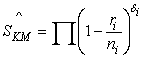(1)

where ni is the number of individuals who are alive just before time Ti, including those who are about to die at this time, and ri denotes the number dieing at this time. Kaplan and Meier used Greenwood  variance estimator for their survival function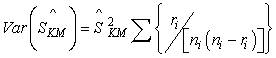(2)

Just like Kaplan-Meier survival function, also Greenwood variance estimator does not consider the waiting time between events.

Exact waiting time survival function

Due to not paying any attention to waiting times between two consecutive events, we assume severity of diseases to be equal. If the waiting time of the occurrence of two events of disease A is always less than that of two events of disease B, disease A is assumed to be more severe than B and it's survival probability therefore should be less than the survival probability of disease B. However, when applying conventional Kaplan-Meier survival function we erroneous obtain same survival probabilities for both diseases. To overcome this problem we developed an exact waiting time survival function, assuming the following sequence of observed survival and censored times:

Starting time __________ Event __________ Event _________ End of the study

Let Δi denote the waiting time between two consecutive events with i = 2,3,.,n and Δ1 = 1. We proceed as Δ2 equalling to the waiting time between the first and second event and Δ3 equalling to the time difference between the second and the third event. So on Δn denotes the time difference between the (n-1)th and nth event if the data is free from censoring. If the data consists of censored observations the number of differences is less than the number of observed times. Δ depends on the occurrence of events and Kaplan-Meier survival function gives the probabilities of these events by using the number of events and the number of persons at risk. As events are important parts of survival analysis and Δ is depending on events, Δ is also an essential part of survival analysis. Moreover Δ's also effect survival probabilities, since with passage of time, the number of person's at risk and Δ (not always Δ=1) varies. As Δi and ni's are related (large Δi (>1) and large ni result in a higher survival probability), their product niΔi leads to corresponding results in terms of survival probabilities, but contains much more information about nature and severity of a disease. So, instead of taking ni , we consider niΔi in our exact waiting time survival function and therefore the time difference between two events is directly related to the survival probabilities as well as to the nature of disease, meaning that a larger Δ results in a higher survival probability (indicating the less severity of disease) and vice versa. This concept is not incorporated in the conventional method of Kaplan-Meier survival function. However, if the difference between two consecutive events is 1, niΔi reduces to ni and gives the same survival probability at time i, as that obtained by Kaplan-Meier survival function.

The basic layout of the above introduced procedure, considering discrete survival times, is shown in (Table 2).

By using the Product-Limit procedure the exact waiting time survival function is given by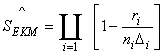(3)

Just like Kaplan-Meier survival function, our exact waiting time function starts from 1 at time zero and it approaches the lower limit 0 if the last observed time is event and the last observed difference equals Δ = 1. Otherwise, it is greater than 0. Unlike the conventional range of Survival function, the range of our proposed estimator is defined by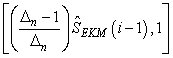If the nth difference [Δn] of our proposed estimator is 1, the range reduces to the range of Kaplan-Meier survival function. However, if this difference is greater than 1, the lower limit of our proposed estimator will always be greater than 0. In this case, our estimator approaches to the form of shrunken estimator. The idea of Shrunken has beneficial in improving the confidence interval coverage rate. The concept is not new in Survival analysis; for example, Parzen  proposed a 'shifted piecewise linear' empirical quantile function and Borkowf  proposed a Shrunken Survival estimator with the range [(2n)-1, 1-(2n)-1]. Due to the behaviour of our new estimator, its lower confidence limits have higher coverage rates than Kaplan-Meier.

Variance of exact waiting time survival function

The variance estimator for our new procedure is obtained by using the delta method. By definition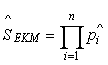(4)

Taking log on both sides,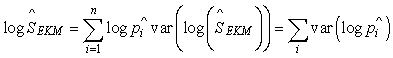(5)

By applying delta method we derive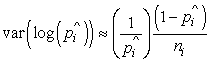and so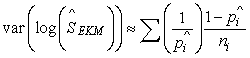(6)

Now applying the delta method a second time on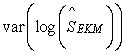we get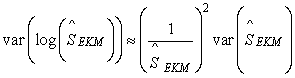And by simplifying we derive our new variance estimator formula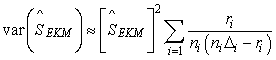(7)

Unlike Greenwood's and Peto's  variance estimators, our new variance estimator uses the exact waiting time between two consecutive events. However, if the data is free from censoring and the differences of times between events equals 1, the new variance is reduced to binomial variance.

Simulation study

In order to compare the performance of our exact waiting time survival function with Kaplan-Meier survival function, we designed three different simulation studies. First, we compared Kaplan-Meier survival function with exact waiting time survival function in terms of Pitman Closeness Criterion; second, we compared confidence interval coverage rates; and third, the width of confidence intervals between both methods.

Comparison of exact waiting time survival function and kaplan-meier survival function by pitman closeness criterion

The Pitman Closeness Criterion  for two estimators at the selected points is defined as PCC = P{EKM - dt = KM - dt}

where dt's denote the deciles of the distribution at t. The criterion states that the absolute error of exact waiting time survival function is smaller than that of Kaplan-Meier survival function if PCC> 0.5. We used the uniform distribution U (0, b) for generating censored survival times. As survival distributions, we choose (1) exponential, (2) weibull (with shape parameter 1.5, 0.5 i.e. monotone increasing and decreasing hazard rate) and (3) log-logistic (different shape parameter with monotone increasing, constant and decreasing hazard rate) distributions.

For comparison, we choose points of the form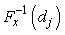with fixed dj's (d1=0.1, d3=.03, d5=0.5, d7=0.7, d9=0.9) and generated 5000 data sets of survival times with various sample sizes (n=15, 50, 100). For each data set of survival times, the censoring times were generated from the uniform distribution. The observed survival times have been obtained from the censored and survival times. In order to get discrete times, we considered the zero decimal points. Pitman Closeness Criterion was calculated for each data set.

Table 3 and Figure 1 illustrate the results of our simulation study. At zero percent censoring, for all five deciles and sample sizes, the criterion gives results in favour of exact waiting time survival function. Also, by increasing censoring percentages, we observe similar results. Only in very few points exact waiting time survival function gives more PCC-error than traditional Kaplan-Meier method. The same situation can be observed from (Figure 1) with selected sample sizes. These results hold for small as well as for large sample sizes and for small to heavy censoring percentages.

Table 3: Pitman Closeness Criterion for various distributions and percent censored at the selected deciles, based on 5000 simulated trials.

Lower confidence limit coverage rate

Considering the same censoring and survival distributions, we inspected the coverage properties of asymptotic normal confidence intervals. By using the estimates of the survival function and standard errors, we construct the asymptotic normal (1-α) 100 percent confidence intervals as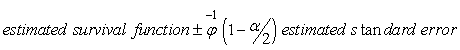We construct the confidence intervals by using Kaplan-Meier survival function and the Greenwood standard error. Similarly, we construct the confidence intervals centred on exact waiting time survival function and our proposed standard error. Since the upper limit of exact waiting time survival function is 1, the lower limit may not approach to the conventional limit i.e. 0. Moreover, as the upper coverage rates give the same results as that obtained by Kaplan-Meier survival function and Greenwood standard error, we are mainly interested in the comparison of the following two coverage rates.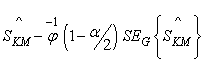(8)

and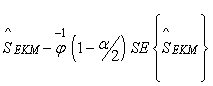(9)

We used the same censoring and survival distributions, but with different percentages of censoring. Table 4 shows the simulated 95% coverage rates of the lower three deciles, as there are more chances of values falling below or equalling to zero. At zero censoring, both methods give the same coverage rate. This may be induced by the fact that the waiting times between events are close to 1. However, with an increasing percentage of censoring, the new method gives better coverage. Only in very few cases and for small censoring percentages, at some points, its coverage rates are smaller than that obtained by the traditional methods

Table 4: Simulated asymptotic Normal 95 per cent lower bound coverage rates at the lower three deciles, with survival times generated from various distributions and censoring times from uniform distribution.

Width of confidence limits

A further simulation study was performed in order to examine the width of the intervals for the same censoring and survival distributions. We calculated the width for both methods by using the simple logic "Upper limit - lower limit" for each simulation and took the sum over all simulations. The lower the width, the better the estimators. We considered the lower three deciles (d1, d2, d3) and the upper three deciles (d7, d8, d9), as in case of very heavy censoring, the lower deciles and their standard errors in most cases are undefined. Therefore, we also included the upper deciles to reach a general decision of the overall performance of both methods. The results of our simulation are summarized in (Table 5). In censored free data, both methods gave the same results, but once again, as censoring increases, our new estimators give much smaller width than Kaplan-Meier and Greenwood standard error.

Table 5: Simulated table for the sum of three lower and three upper deciles, with data generated from various distributions.

Application to a lung cancer data

We compared Kaplan-Meier survival function and exact waiting time survival function on a data set from the Veterans Administration lung cancer trial (presented by Prentice  and also used by Gupta ), where chemotherapy was induced to males with advanced inoperable lung cancer. For convenience, we considered the same part of the data set that Gupta used in his paper, consisting of 97 patients out of which 91 were events and 6 were censored. The survival times, given in days, were as following:

 72 228 10 110 314 100* 42 144 30 384 4 13 123* 97* 59 117 151 22 18 139 20 31 52 18 51 122 27 54 7 63 392 92 35 117 132 162 3 95 162 216 553 278 260 156 182* 143 105 103 112 87* 242 111 587 389 33 25 357 467 1 30 283 25 21 13 87 7 24 99 8 99 61 25 95 80 29 24 83* 31 51 52 73 8 36 48 7 140 186 19 45 80 52 53 15 133 111 378 49

* denotes a censored observation.

Table 6 (included as supplementary data) summarizes the data and methods in 11 columns, where column 1 shows the time in days, column 2 the number of person's at risk (ni), followed by the event column 3 (ri). Column 4 shows the number censored (ci) at different times. Δ is the heading of column 5 and represents the waiting time between two consecutive events. As the first Δ is 1, the difference between first and second waiting time is 3-1=2 days, and so on we calculated the other waiting times. The next two columns represent Kaplan-Meier survival function and exact waiting time survival function, whereby both have the same value at first event, i.e. the first observed time. The important role of the concept of waiting times starts at the next stage, as from here both methods give different results in terms of survival probabilities This difference continues to exist through to the end of the analysis. Since the last observed time 587 (days) is an event, Kaplan- Meier survival function gives the value zero, while exact waiting time survival function yields a survival probability at that time equalling to 0.312. This is due to the fact that the waiting time between the last two observed events is greater than 1, i.e. 587-553= 34.

Lower confidence limits of Kaplan-Meier survival function and exact waiting time survival function are shown in columns 8 and 9. Here, we do not round the negative limit to zero, as we want to check the full behaviour of the two methods. We can see from the columns that the lower limit constructed by Kaplan-Meier gives 16 negative limits, while when constructed by exact waiting time survival function all these values are greater than zero. This shows that exact waiting time survival function is a type of left Shrunken Kaplan-Meier survival function and gives better results at this end. The next two columns give the upper limits of both methods and again we give the full estimates. In case of Kaplan-Meier survival function, there are 3 values greater than 1, while our exact waiting time survival function gives 4 values, due to a higher survival probability by this method at that point. Except this, the other limits settle down to routine. The last two columns give the widths of intervals for the two methods. We can see that at each time the width of confidence intervals constructed by exact waiting time survival function is smaller than that obtained by Kaplan-Meier survival function. These findings are also shown in (Figures 2 and 3).

#### Discussion

Kaplan-Meier survival function, which is a non-parametric technique of survival analysis, remains a reliable and frequently used method in medical research, as it is easy to understand, calculate and interpret. For the construction of confidence intervals, Greenwood variance estimator is commonly used. However, they both share the drawback of giving same results for two diseases of different nature, by ignoring waiting times between two consecutive events. In light of this deficiency, in this paper we proposed an exact waiting time survival function and a modified variance estimator. Unlike the traditional methods, these new estimators explicitly consider the waiting time between two events. The new methods perform equally well for both, small and large sample sizes. Similarly, as censoring increases, the performance of our new estimators increases as well. The new estimators give better coverage rates for the lower confidence limits and yield in considerably smaller width of confidence intervals. Finally, the simplicity of theses estimators make them attractive for use in different fields of medical research.

#### References

Select your language of interest to view the total content in your interested language

### Article Usage

• Total views: 12192
• [From(publication date):
September-2011 - Dec 14, 2019]
• Breakdown by view type
• HTML page views : 8384Can't read the image? click here to refresh